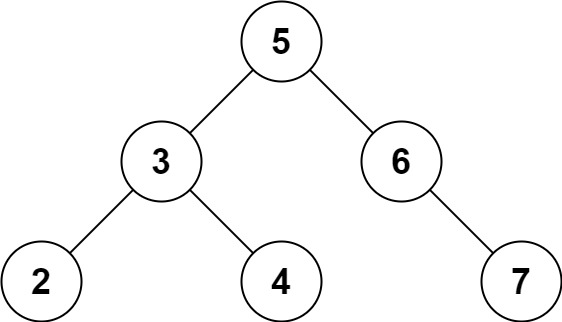## 653. Two Sum IV - Input is a BST

Given the `root` of a Binary Search Tree and a target number `k`, return `true` if there exist two elements in the BST such that their sum is equal to the given target.

Example 1:```Input: root = [5,3,6,2,4,null,7], k = 9
Output: true
```

Example 2:```Input: root = [5,3,6,2,4,null,7], k = 28
Output: false
```

Example 3:

```Input: root = [2,1,3], k = 4
Output: true
```

Example 4:

```Input: root = [2,1,3], k = 1
Output: false
```

Example 5:

```Input: root = [2,1,3], k = 3
Output: true
```

Constraints:

• The number of nodes in the tree is in the range `[1, 104]`.
• `-104 <= Node.val <= 104`
• `root` is guaranteed to be a valid binary search tree.
• `-105 <= k <= 105`

## Rust Solution

``````struct Solution;
use rustgym_util::*;
use std::cmp::Ordering::*;

trait Inorder {
fn inorder(&self, v: &mut Vec<i32>, target: i32);
}

fn inorder(&self, v: &mut Vec<i32>, target: i32) {
if let Some(node) = self {
let node = node.borrow();
let left = &node.left;
let right = &node.right;
Self::inorder(left, v, target);
v.push(node.val);
Self::inorder(right, v, target);
}
}
}

impl Solution {
fn find_target(root: TreeLink, k: i32) -> bool {
let mut v = vec![];
root.inorder(&mut v, k);
let n = v.len();
let mut l = 0;
let mut r = n - 1;
while l < r {
let sum = v[l] + v[r];
match sum.cmp(&k) {
Greater => {
r -= 1;
}
Less => {
l += 1;
}
Equal => {
return true;
}
}
}
false
}
}

#[test]
fn test() {
let root = tree!(5, tree!(3, tree!(2), tree!(4)), tree!(6, None, tree!(7)));
assert_eq!(Solution::find_target(root, 9), true);
}
``````

Having problems with this solution? Click here to submit an issue on github.